# RS Aggarwal Solutions for Class 10 Maths Chapter 17 Volume and Surface Area of Solids Exercise 17C

R S Aggarwal solutions are given here contain answers to all the questions enlisted in the exercise 17C. Practice R S Aggarwal Maths Chapter 17 for Class 10 effectively to score good marks in the examinations. This exercise helps students to solve problems on Volume and Surface Area of a frustum of a cone and its derivation. At the end of the exercise, students will be able to solve any problem based on the concept.

## Download PDF of RS Aggarwal Solutions for Class 10 Maths Chapter 17 Volume and Surface Area of Solids Exercise 17C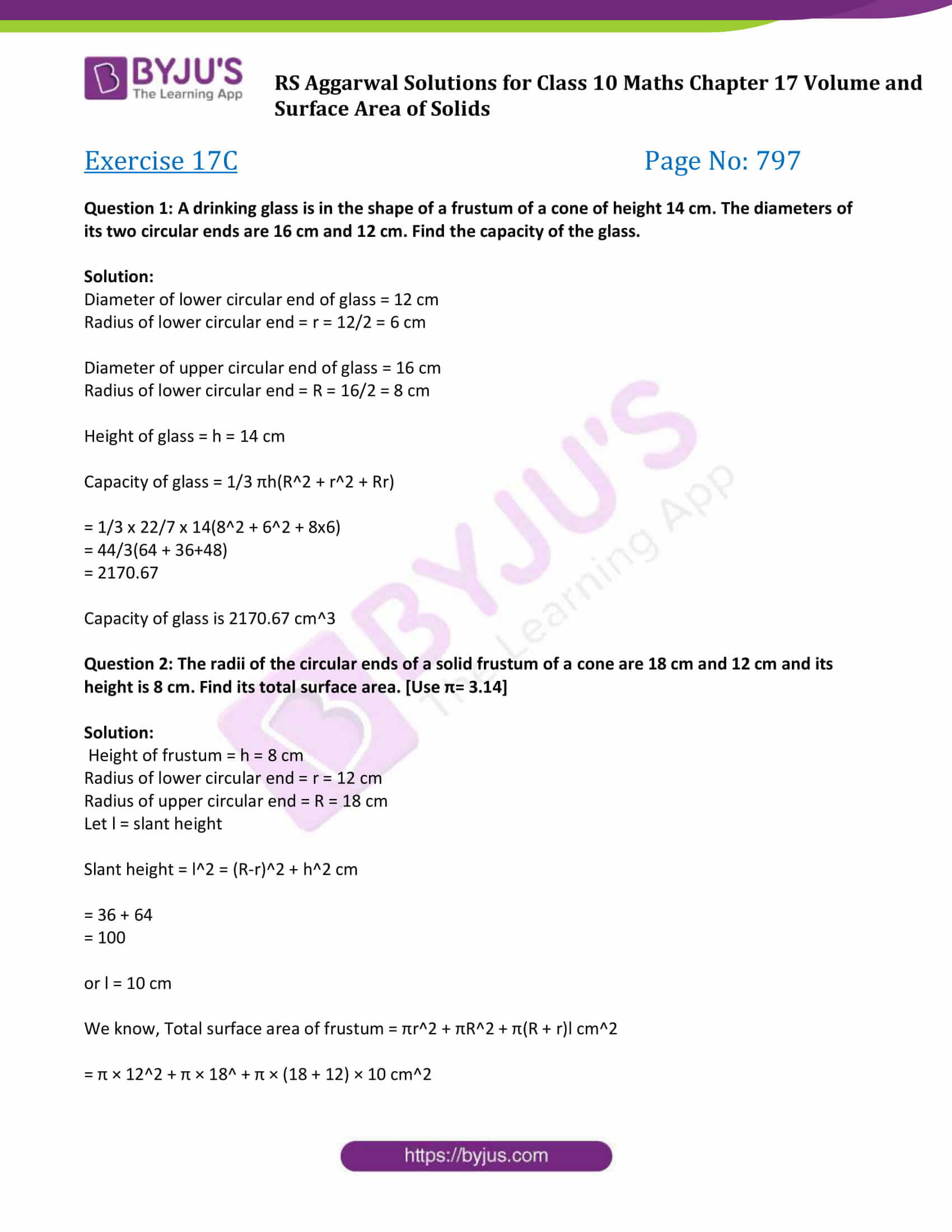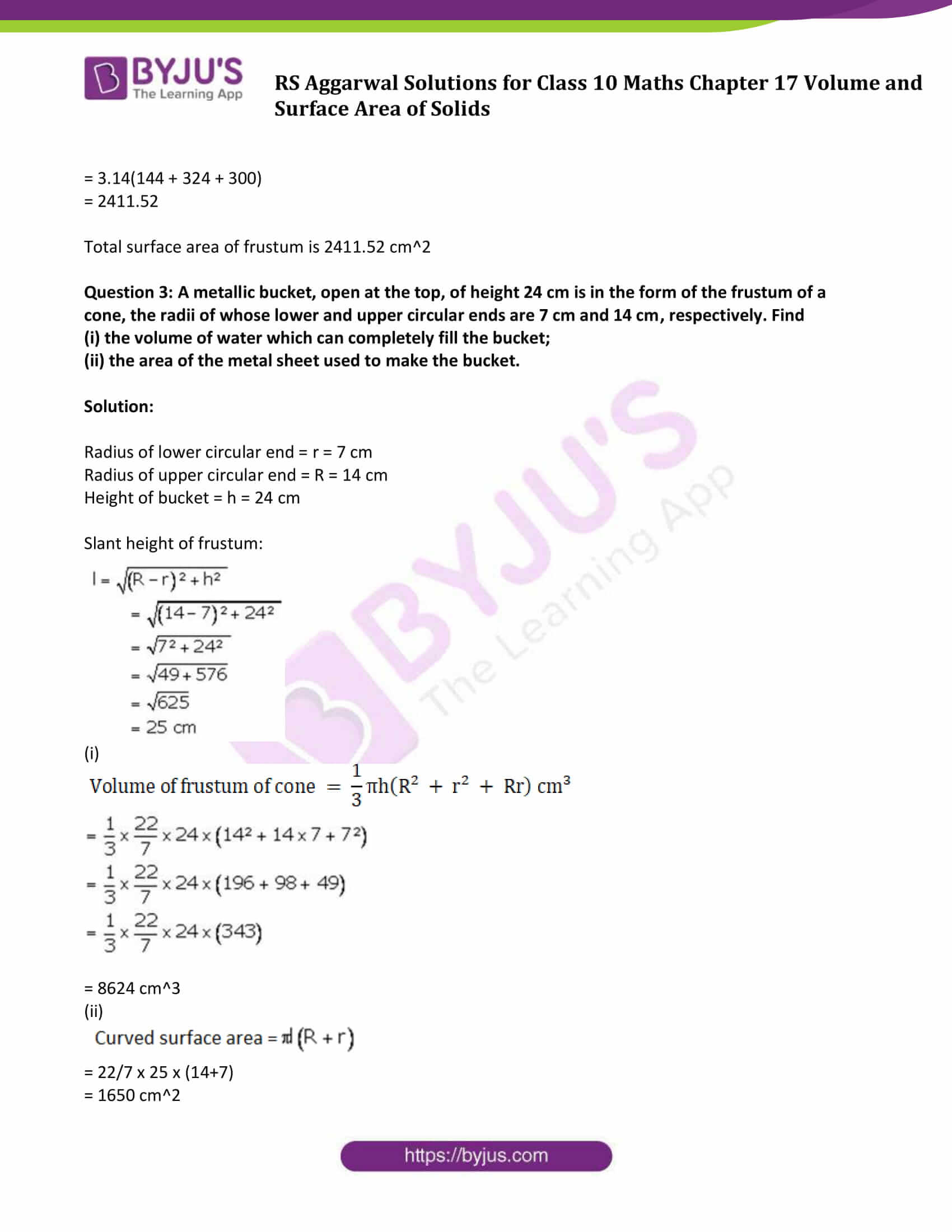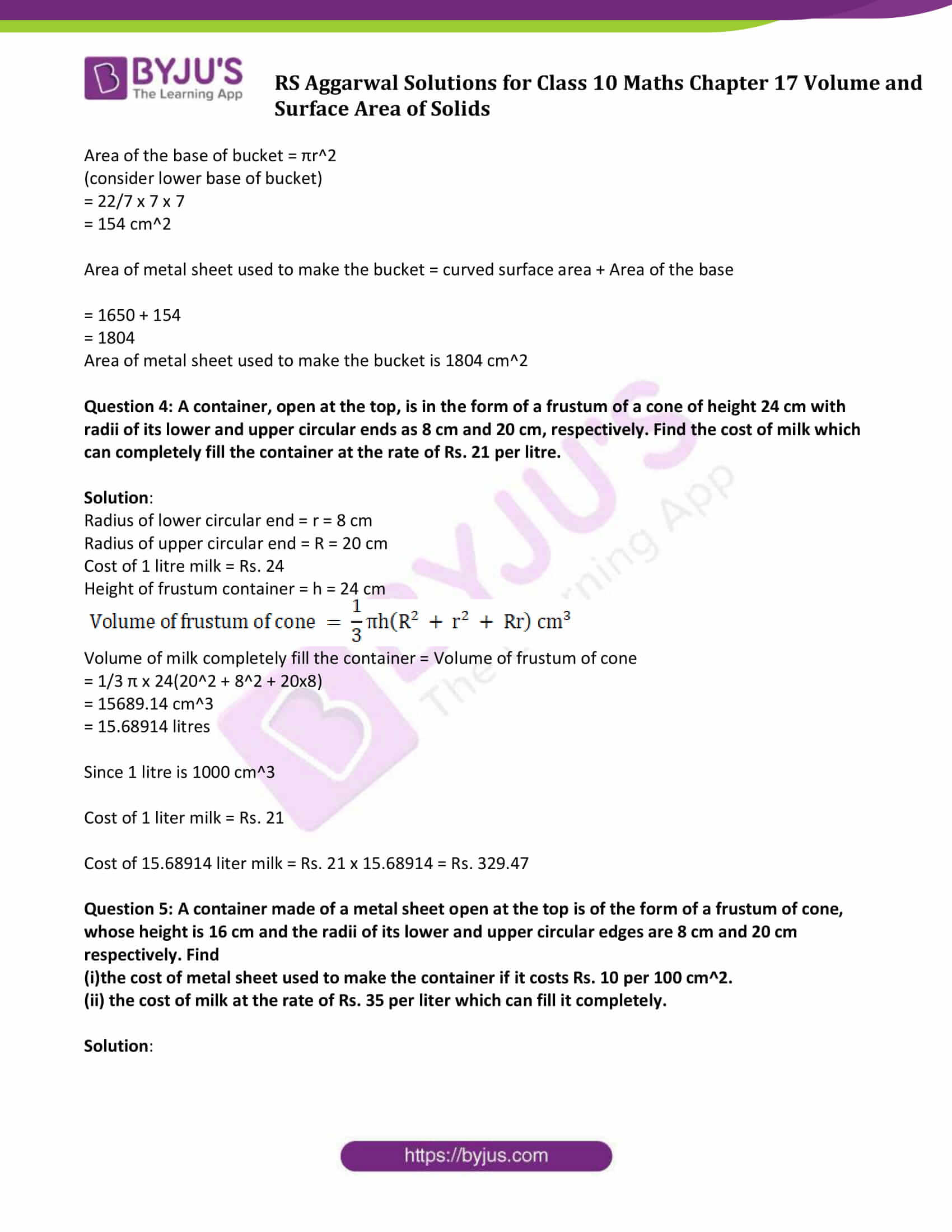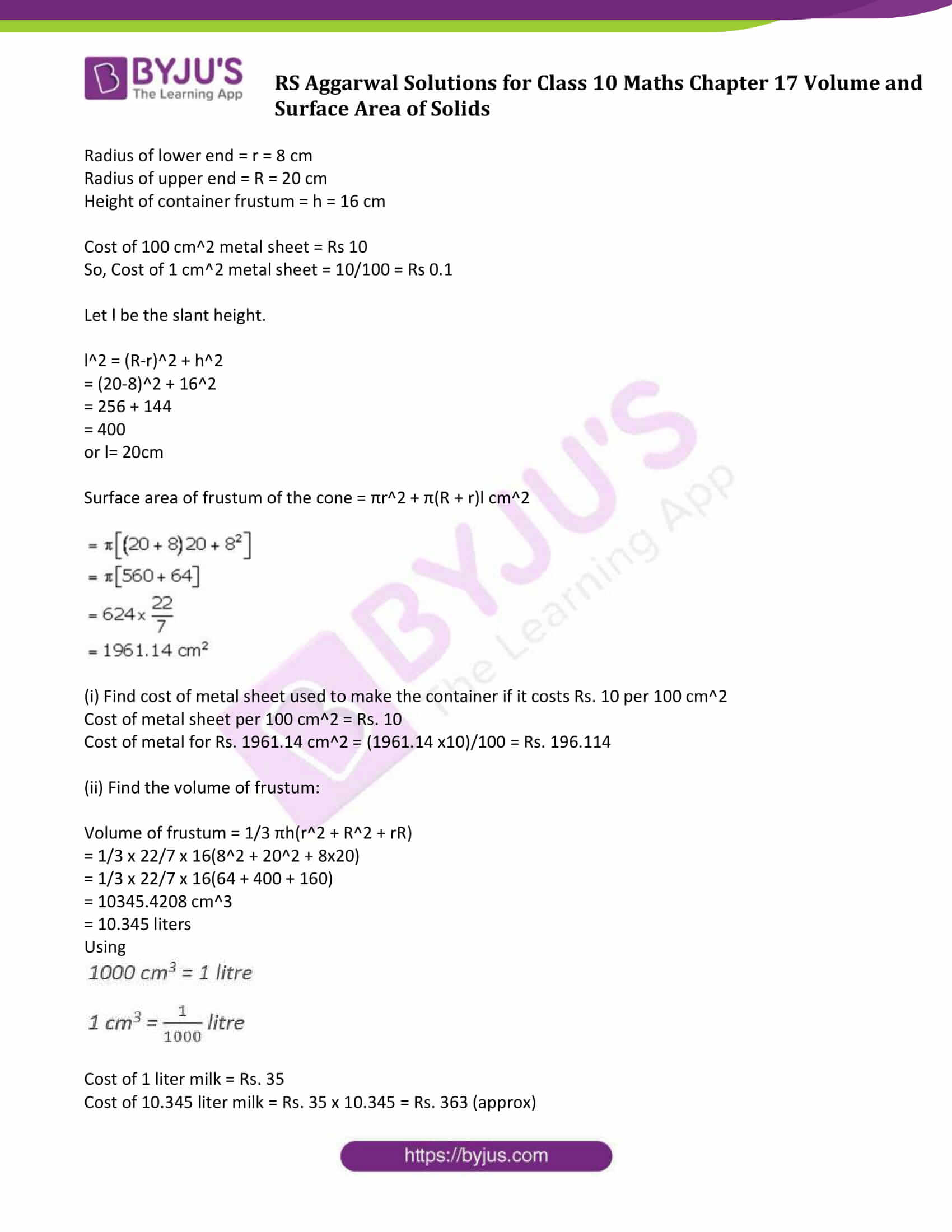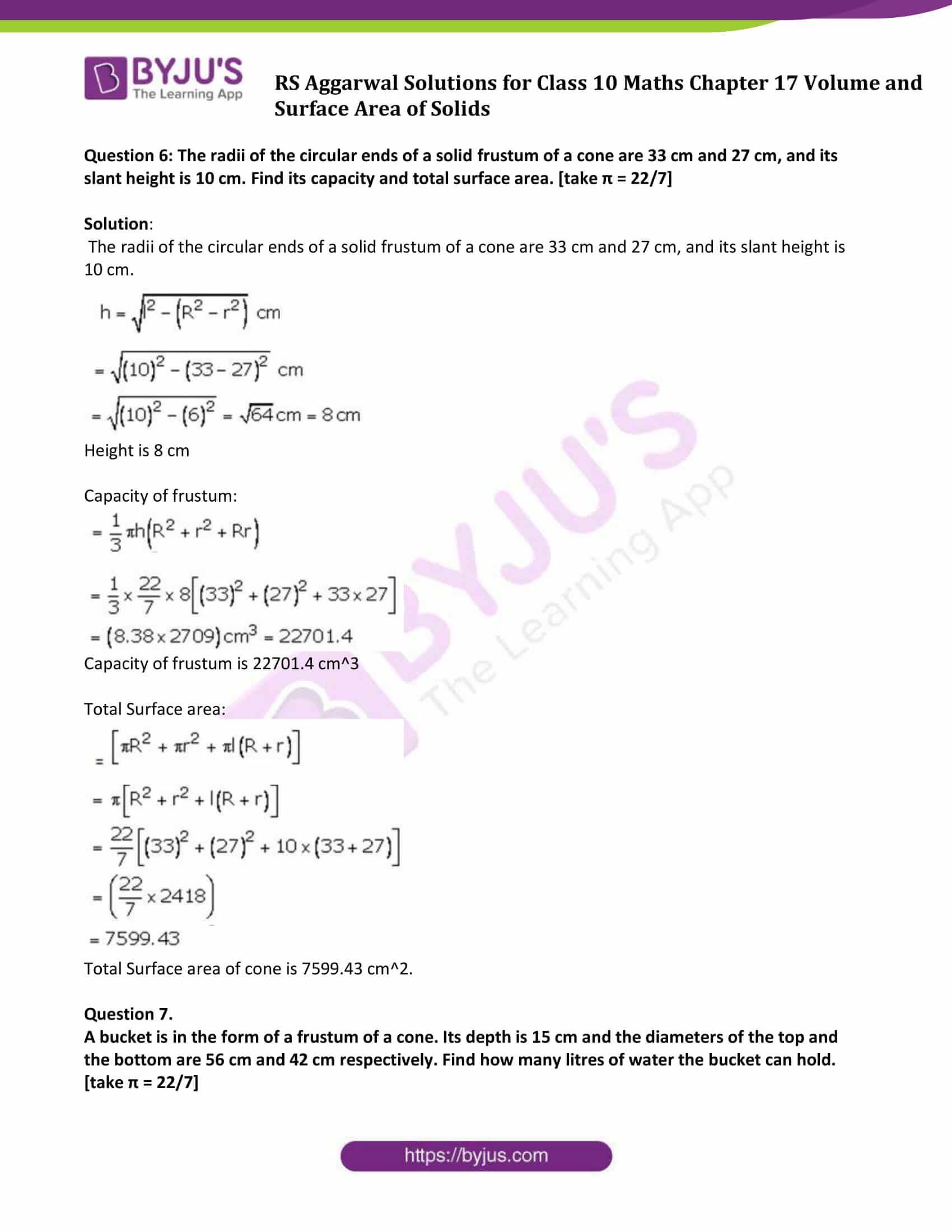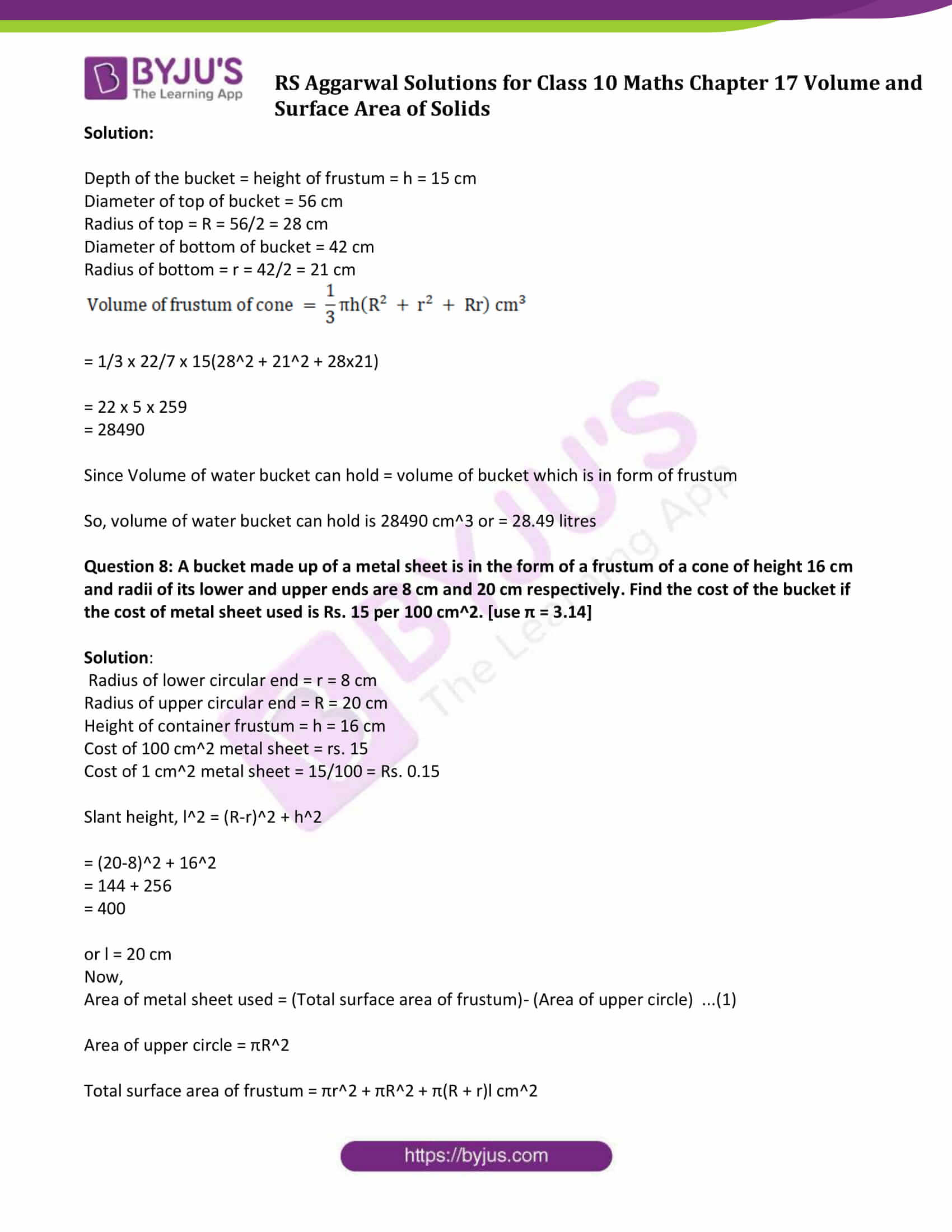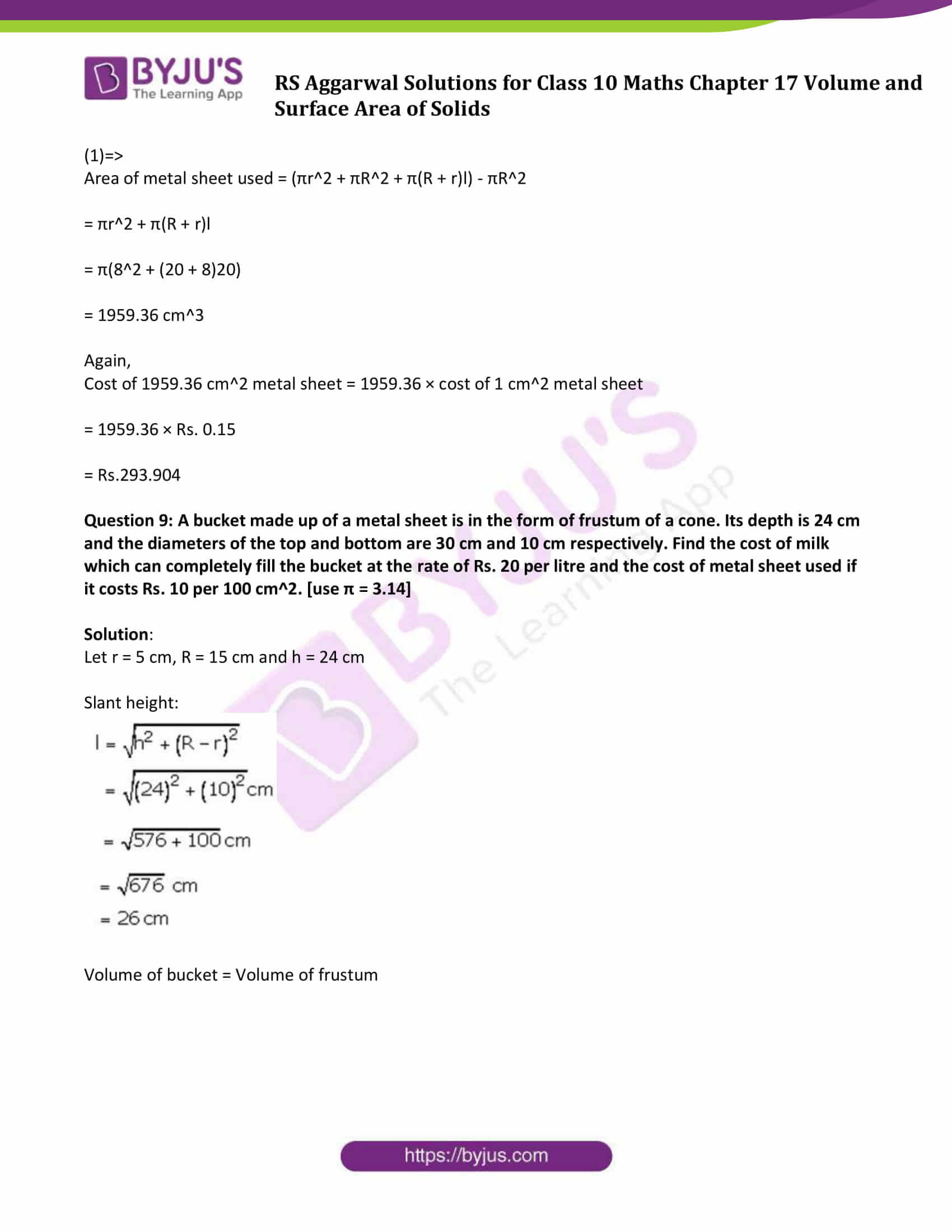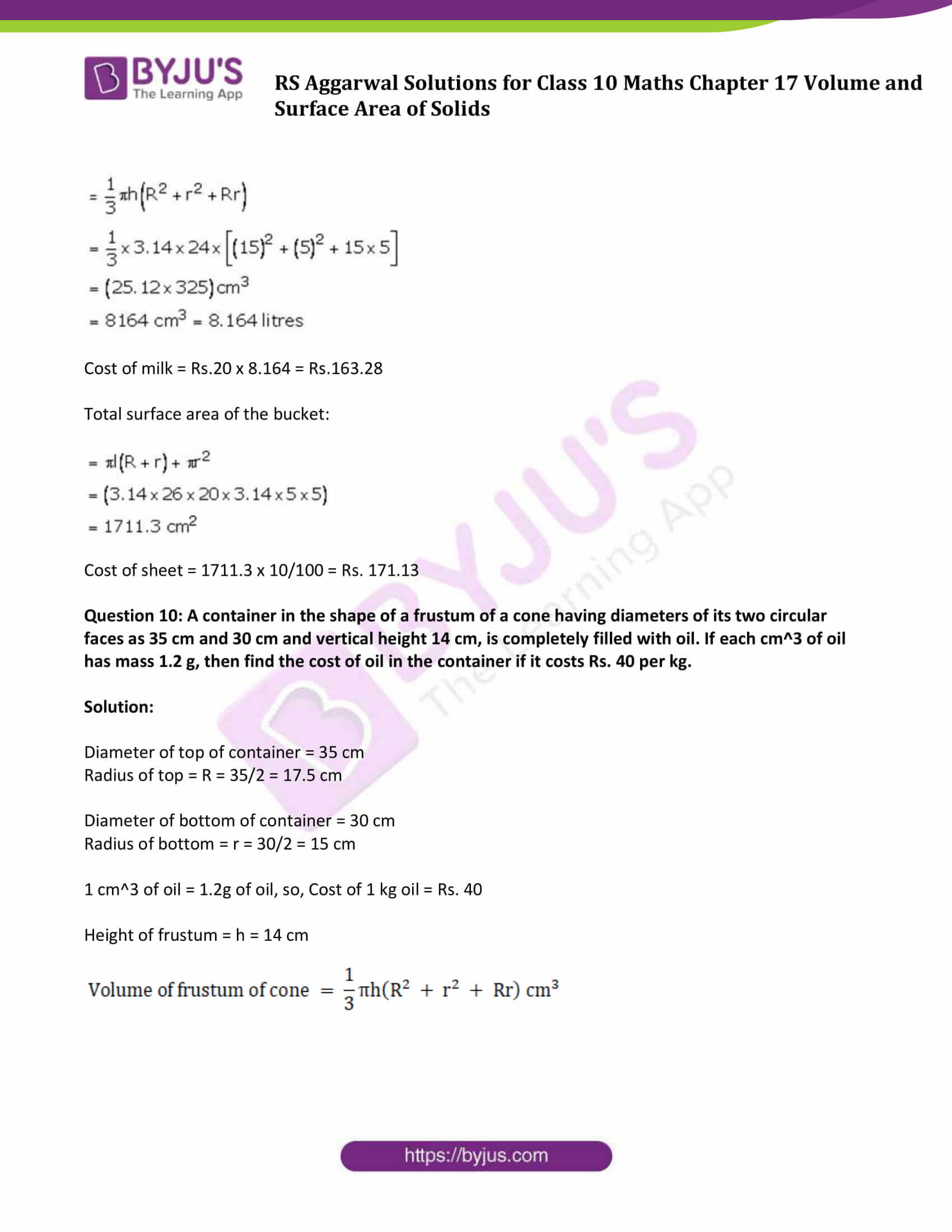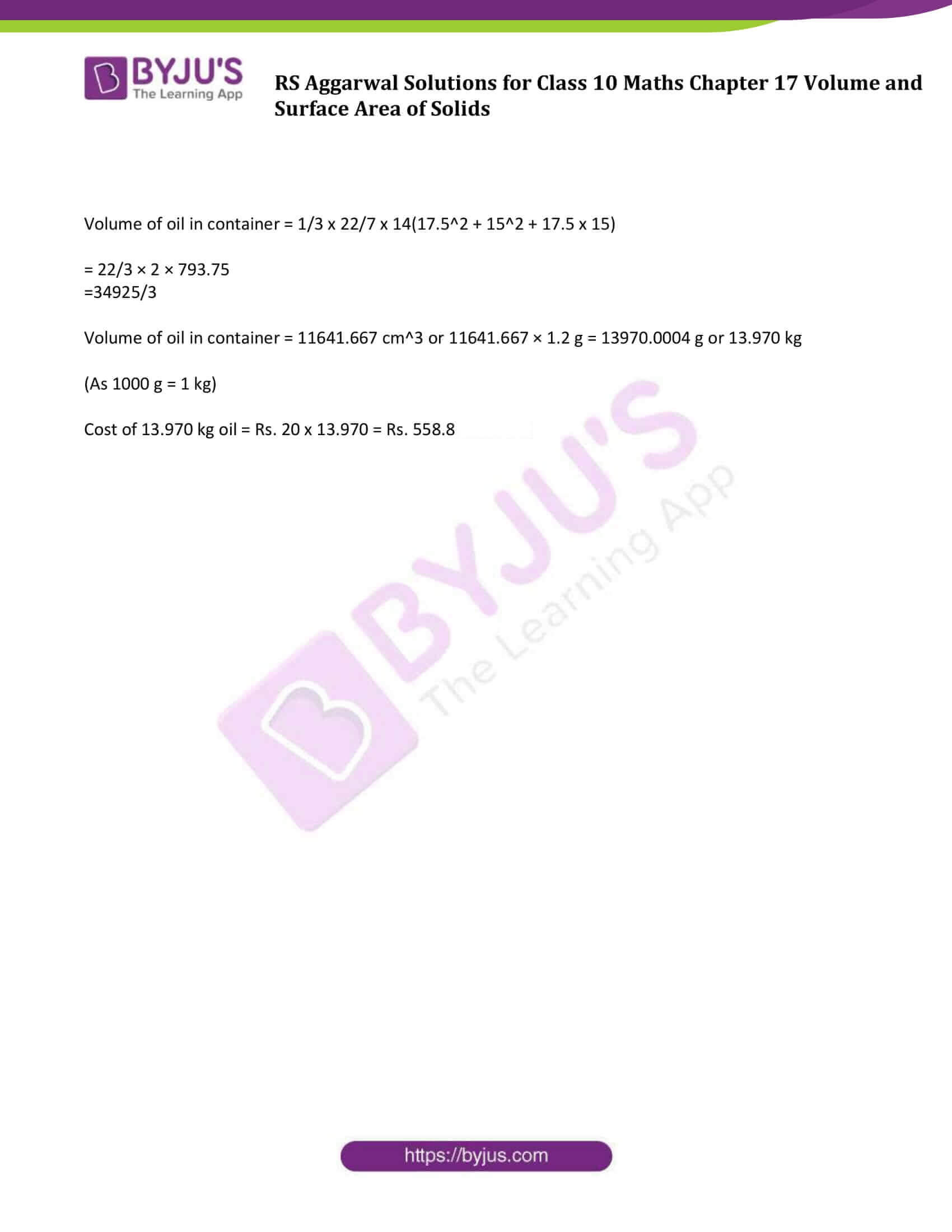### Access other exercise solutions of Class 10 Maths Chapter 17 Volume and Surface Area of Solids

Exercise 17 A Solutions

Exercise 17 B Solutions

Exercise 17 D Solutions

### Access Solutions of Maths RS Aggarwal Class 10 Chapter 17 Volume and Surface Area of Solids Exercise 17C

Question 1: A drinking glass is in the shape of a frustum of a cone of height 14 cm. The diameters of its two circular ends are 16 cm and 12 cm. Find the capacity of the glass.

Solution:

Diameter of lower circular end of glass = 12 cm

Radius of lower circular end = r = 12/2 = 6 cm

Diameter of upper circular end of glass = 16 cm

Radius of lower circular end = R = 16/2 = 8 cm

Height of glass = h = 14 cm

Capacity of glass = 1/3 πh(R2 + r2 + Rr)

= 1/3 x 22/7 x 14(82 + 62 + 8×6)

= 44/3(64 + 36+48)

= 2170.67

Capacity of glass is 2170.67 cm3

Question 2: The radii of the circular ends of a solid frustum of a cone are 18 cm and 12 cm and its height is 8 cm. Find its total surface area. [Use π= 3.14]

Solution:

Height of frustum = h = 8 cm

Radius of lower circular end = r = 12 cm

Radius of upper circular end = R = 18 cm

Let l = slant height

Slant height = l2 = (R-r)2 + h2 cm

= 36 + 64

= 100

or l = 10 cm

We know, Total surface area of frustum = πr2 + πR2 + π(R + r)l cm2

= π × 122 + π × 18^ + π × (18 + 12) × 10 cm2

= 3.14(144 + 324 + 300)

= 2411.52

Total surface area of frustum is 2411.52 cm2

Question 3: A metallic bucket, open at the top, of height 24 cm is in the form of the frustum of a cone, the radii of whose lower and upper circular ends are 7 cm and 14 cm, respectively. Find

(i) the volume of water which can completely fill the bucket;

(ii) the area of the metal sheet used to make the bucket.

Solution:

Radius of lower circular end = r = 7 cm

Radius of upper circular end = R = 14 cm

Height of bucket = h = 24 cm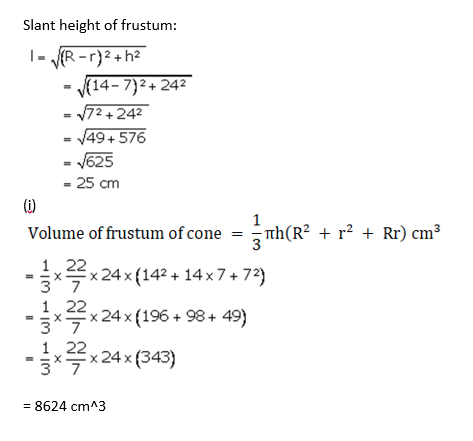(ii)Curved surface area = πl( R+r)

= 22/7 x 25 x (14+7)

= 1650 cm2

Area of the base of bucket = πr2

(consider lower base of bucket)

= 22/7 x 7 x 7

= 154 cm2

Area of metal sheet used to make the bucket = curved surface area + Area of the base

= 1650 + 154

= 1804

Area of metal sheet used to make the bucket is 1804 cm2

Question 4: A container, open at the top, is in the form of a frustum of a cone of height 24 cm with radii of its lower and upper circular ends as 8 cm and 20 cm, respectively. Find the cost of milk which can completely fill the container at the rate of Rs. 21 per litre.

Solution:

Radius of lower circular end = r = 8 cm

Radius of upper circular end = R = 20 cm

Cost of 1 litre milk = Rs. 24

Height of frustum container = h = 24 cm

Volume of frustum of cone = 1/3π h(R2 + r2 + Rr) cm3

Volume of milk completely fill the container = Volume of frustum of cone

= 1/3 π x 24(202 + 82 + 20×8)

= 15689.14 cm3

= 15.68914 litres

Since 1 litre is 1000 cm3

Cost of 1 liter milk = Rs. 21

Cost of 15.68914 liter milk = Rs. 21 x 15.68914 = Rs. 329.47

Question 5: A container made of a metal sheet open at the top is of the form of a frustum of cone, whose height is 16 cm and the radii of its lower and upper circular edges are 8 cm and 20 cm respectively. Find

(i)the cost of metal sheet used to make the container if it costs Rs. 10 per 100 cm2.

(ii) the cost of milk at the rate of Rs. 35 per liter which can fill it completely.

Solution:

Radius of lower end = r = 8 cm

Radius of upper end = R = 20 cm

Height of container frustum = h = 16 cm

Cost of 100 cm2 metal sheet = Rs 10

So, Cost of 1 cm2 metal sheet = 10/100 = Rs 0.1

Let l be the slant height.

l2 = (R-r)2 + h2

= (20-8)2 + 162

= 256 + 144

= 400

or l= 20cm

Surface area of frustum of the cone = πr2 + π(R + r)l cm2

= π[ (20 + 8 )20 + 82]

= π[560 + 64]

= 624 X ( 22/7 )

= 1961.14 cm2

(i) Find cost of metal sheet used to make the container if it costs Rs. 10 per 100 cm2

Cost of metal sheet per 100 cm2 = Rs. 10

Cost of metal for Rs. 1961.14 cm2 = (1961.14 x10)/100 = Rs. 196.114

(ii) Find the volume of frustum:

Volume of frustum = 1/3 πh(r2 + R2 + rR)

= 1/3 x 22/7 x 16(82 + 202 + 8×20)

= 1/3 x 22/7 x 16(64 + 400 + 160)

= 10345.4208 cm3

= 10.345 liters

Using

1000cm3 = 1 litre

1 cm3 = 1/1000 litre

Cost of 1 liter milk = Rs. 35

Cost of 10.345 liter milk = Rs. 35 x 10.345 = Rs. 363 (approx)

Question 6: The radii of the circular ends of a solid frustum of a cone are 33 cm and 27 cm, and its slant height is 10 cm. Find its capacity and total surface area. [take π = 22/7]

Solution: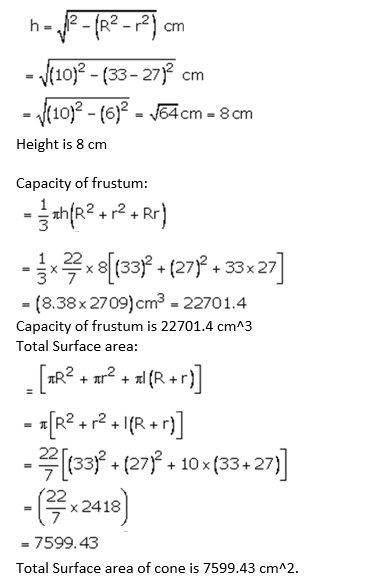Question 7.

A bucket is in the form of a frustum of a cone. Its depth is 15 cm and the diameters of the top and the bottom are 56 cm and 42 cm respectively. Find how many litres of water the bucket can hold. [take π = 22/7]

Solution:

Depth of the bucket = height of frustum = h = 15 cm

Diameter of top of bucket = 56 cm

Radius of top = R = 56/2 = 28 cm

Diameter of bottom of bucket = 42 cm

Radius of bottom = r = 42/2 = 21 cm

Volume of frustum of cone = 1/3π h(R2 + r2 + Rr) cm3

= 1/3 x 22/7 x 15(282 + 212 + 28×21)

= 22 x 5 x 259

= 28490

Since Volume of water bucket can hold = volume of bucket which is in form of frustum

So, volume of water bucket can hold is 28490 cm3 or = 28.49 litres

Question 8: A bucket made up of a metal sheet is in the form of a frustum of a cone of height 16 cm and radii of its lower and upper ends are 8 cm and 20 cm respectively. Find the cost of the bucket if the cost of metal sheet used is Rs. 15 per 100 cm2. [use π = 3.14]

Solution:

Radius of lower circular end = r = 8 cm

Radius of upper circular end = R = 20 cm

Height of container frustum = h = 16 cm

Cost of 100 cm2 metal sheet = rs. 15

Cost of 1 cm2 metal sheet = 15/100 = Rs. 0.15

Slant height, l2 = (R-r)2 + h2

= (20-8)2 + 162

= 144 + 256

= 400

or l = 20 cm

Now,

Area of metal sheet used = (Total surface area of frustum)- (Area of upper circle) …(1)

Area of upper circle = πR2

Total surface area of frustum = πr2 + πR2 + π(R + r)l cm2

(1)⇨

Area of metal sheet used = (πr2 + πR2 + π(R + r)l) – πR2

= πr2 + π(R + r)l

= π(82 + (20 + 8)20)

= 1959.36 cm3

Again,

Cost of 1959.36 cm2 metal sheet = 1959.36 × cost of 1 cm2 metal sheet

= 1959.36 × Rs. 0.15

= Rs.293.904

Question 9: A bucket made up of a metal sheet is in the form of frustum of a cone. Its depth is 24 cm and the diameters of the top and bottom are 30 cm and 10 cm respectively. Find the cost of milk which can completely fill the bucket at the rate of Rs. 20 per litre and the cost of metal sheet used if it costs Rs. 10 per 100 cm2. [use π = 3.14]

Solution:

Let r = 5 cm, R = 15 cm and h = 24 cm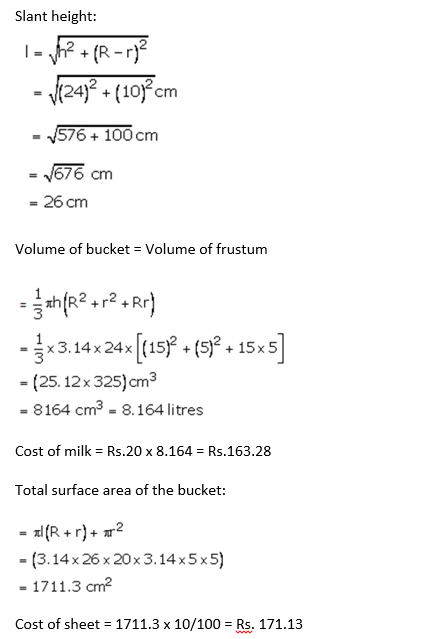Question 10: A container in the shape of a frustum of a cone having diameters of its two circular faces as 35 cm and 30 cm and vertical height 14 cm, is completely filled with oil. If each cm3 of oil has mass 1.2 g, then find the cost of oil in the container if it costs Rs. 40 per kg.

Solution:

Diameter of top of container = 35 cm

Radius of top = R = 35/2 = 17.5 cm

Diameter of bottom of container = 30 cm

Radius of bottom = r = 30/2 = 15 cm

1 cm3 of oil = 1.2g of oil, so, Cost of 1 kg oil = Rs. 40

Height of frustum = h = 14 cm

Volume of frustum of cone = 1/3π h(R2 + r2 + Rr) cm3

Volume of oil in container = 1/3 x 22/7 x 14(17.52 + 152 + 17.5 x 15)

= 22/3 × 2 × 793.75

=34925/3

Volume of oil in container = 11641.667 cm3 or 11641.667 × 1.2 g = 13970.0004 g or 13.970 kg

(As 1000 g = 1 kg)

Cost of 13.970 kg oil = Rs. 20 x 13.970 = Rs. 558.8

## RS Aggarwal Solutions for Class 10 Maths Chapter 17 Volume and Surface Area of Solids Exercise 17C Topics:

Class 10 Maths Chapter 17 Volume and Surface Area of Solids Exercise 17C is based on the topics:

• Volume and Surface Area of a frustum of a cone
• Derivation of Volume and Surface Area of a frustum of a cone